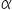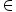Maths-
General
Easy

Question

# Let F() =, whereR. Then (F())–1 is equal to

## The correct answer is: F (–)

### We are asked to find the value of [F(α)] inverseTherefore the correct option is choice 1#### With Turito Foundation.#### Get an Expert Advice From Turito.